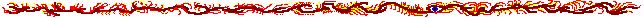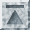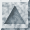# mandel.cc [plain] [printer]

```#include "mpi++.h"
#include "mpe++.h"
#include "mpistream.h"

#include <stdio.h>
#include <applic.h>
#include <unistd.h>
#include <Math.h>
#include <complex.h>

// We use the standard C++ complex value implementation
typedef complex<double> Complex;

int mandel (const Complex& c) {
Complex z (0.0, 0.0);
int count   = 0,
max     = 255; // Max nr of iterations
double len2;

/* Mandelbrot function */
do {
z = z*z + c;
len2 = sqr(z.real()) + sqr(z.imag());
} while ( len2<4.0 && ++count<max );

return count;
}

Main () {
MPIInstance mpi (mArgc, mArgv);
MPIIOStream mpis (mpi);

int resX = 400,
resY = 400;

// We use only the default colors (strange, there didn't seem to
// exist a method to query the color handles in the MPE...)
MPE_Color defcolors[] = {MPE_WHITE, MPE_RED, MPE_YELLOW, MPE_GREEN,
MPE_CYAN, MPE_BLUE, MPE_MAGENTA, MPE_AQUAMARINE, MPE_FORESTGREEN, MPE_ORANGE,
MPE_MAROON, MPE_BROWN, MPE_PINK, MPE_CORAL, MPE_GRAY, MPE_BLACK};

try {
if (mpi.world().getRank() == 0) {
//
//  Master
//

// Open graphics
MPEWindow win (mpi.world(), 0,0, resX,resY, NULL);
fprintf (stderr, "Colors=%d\n", win.colors ());

// This update() is a bug workaround.
// There was a very strange ''feature'' in the program:
// after the initial image had been drawn, and the
// MPI_Get_drag_region() was called, the window was
// wiped clean (all white). This didn't occur after
// zooming in on smaller areas- I really can't understand what
// could have caused it, because the only drawing operations are
// the pixel drawing done in the slaves. It didn't behave
// like this earlier, when I made all the calculations and
// drawing in the master. This update seems to fix it.
win.update ();

// The main loop draws fractals, gets input from the user, and
// then draws fractals according to the input
Complex upperleft (-2, -2), lowerright (2,2);
while (true) {
// Measure drawing time
double time1 = mpi.time ();

double imStep = (lowerright.imag()-upperleft.imag())/resY;
double reStep = (lowerright.real()-upperleft.real())/resX;

// Draw the fractal
for (int imrow=0; imrow<resY; imrow++) {
// Determine who processes this row
int slave;
if (imrow < mpi.world().size()-1) {
// Send the first rows to slaves
slave = imrow+1;
} else {
// Then send more stuff to the slave that
// first finishes calculating its previous row
// (The slaves send their rank when they finish)
slave = int (mpi.world().recv (100, MPI_ANY_SOURCE));
}

// Send the data to the child as a string. A bit
// inefficient, yes, but I haven't had time yet to
// make the sends accept other types than Strings.
mpis.sendTo(slave) << upperleft.real()
<< upperleft.imag()+imStep*imrow
<< imrow
<< reStep;
mpis.flush ();
mpi.world().nbSend(format("%.30g %.30g %d %.30g", upperleft.real(),
upperleft.imag()+imStep*imrow, imrow,
reStep), slave);
}

// Wait for the children to finish drawing...
// (They should all be busy calculating right now)
for (int i=0; i<mpi.world().size()-1; i++)
mpi.world().recv (100, MPI_ANY_SOURCE);

// Print drawing time
double time2 = mpi.time ();
printf ("Time for drawing the frame: %g s\n", time2-time1);
fflush (stdout);

// Select new area
Rectangle<int> rect = win.getDragRegion (MPE_BUTTON1, MPE_DRAG_RECT);

// If the area was very small, terminate execution
if (abs(rect.y1-rect.y2)<10 && abs(rect.x2-rect.x1)<10)
break;

// Find the complex coordinates for the selected region
Complex newUL (upperleft.real()+rect.x1*reStep,
upperleft.imag()+rect.y1*imStep);
Complex newLR (upperleft.real()+rect.x2*reStep,
upperleft.imag()+rect.y2*imStep);
upperleft = newUL;
lowerright = newLR;
}
printf ("Exiting...\n");

// Tell slaves to terminate nicely
for (int i=1; i<mpi.world().size(); i++)
mpi.world().send ("end", i);

// For some strange reason, my libmpe doesn't have the
// MPE_Close function linked in, although it exists in the
// headers. The window closes anyways, so this is not really needed.
// MPE_Close (win);
} else {
//
//  Slave
//

MPEWindow win (mpi.world(), 0,0, resX,resY, NULL);

// Loop until ordered to terminate
while (true) {
// Wait row-drawing command from the master
String buffer = mpi.world().recv (200, 0);

if (buffer == "end")
break;

// Read parameters from the message (they are in string format)
Array<String> pars;
buffer.split (pars, ' '); // Split the string to an array
double reStart  = pars;
double imStart  = pars;
int imRow       = int (pars);
double reStep   = double (pars);

// Draw the row
for (int recol=0; recol<resX; recol++) {
int color = mandel (Complex (reStart+recol*reStep, imStart));
win.drawPoint (recol, imRow, defcolors[color%16]);
}
win.update (); // Actually draw it

// Tell master that we have finished with the row
mpi.world().nbSend (String(mpi.world().getRank()), 0);
}
}
} catch (exception& e) {
// Perhaps the exception control should be done somehow
// otherwise. Now, for example, if the master excepts, the
// slaves will be left hanging, which is not desirable. But,
// of course it doesn't except, because this program has no
// errors.
fprintf (stderr, "MPI/MPE exception caught: %s\n", (CONSTR) e.what());
}
}
```Generated with (Python version) of tab4.cgi by Marko Grönroos (magi@iki.fi), 1999